Question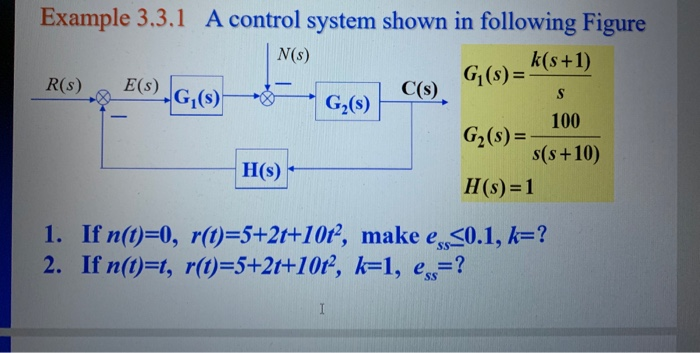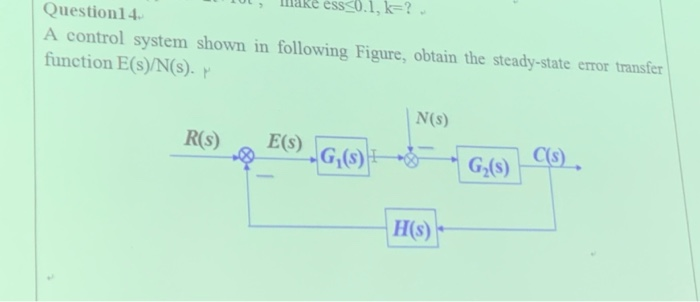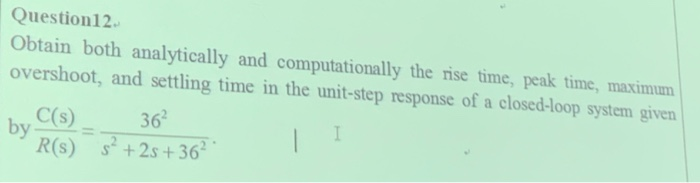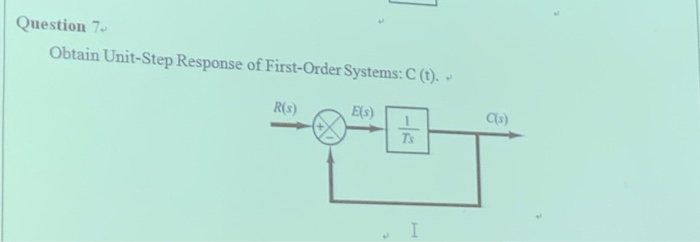Example 3.3.1 A control system shown in following Figure G(s)=(s+1) C(s) N(s) E(s) G,(S) R(s) S G2(s) 100 G2(s)= s(s+10) H(s) H(s) 1 1. If n(t) 0, r(t)=5+2t+10t?, make e 0.1, k-? 2. If n(t)=t, r(t)=5+2t+10t2, k=1, e=? sS I
ess0.1, k=?. Question14 A control system shown in following Figure, obtain the steady-state error transfer function E(s)/N(s). N(s) E(S) GS C(S) G.(S) R(s) H(s)
Question12 Obtain both analytically and computationally the rise time, peak time, maximum overshoot, and settling time in the unit-step response of a closed-loop system given 362 C(s) by R(s) +2s+36
Question 7 Obtain Unit-Step Response of First-Order Systems: C (t). R(s) Els) Ts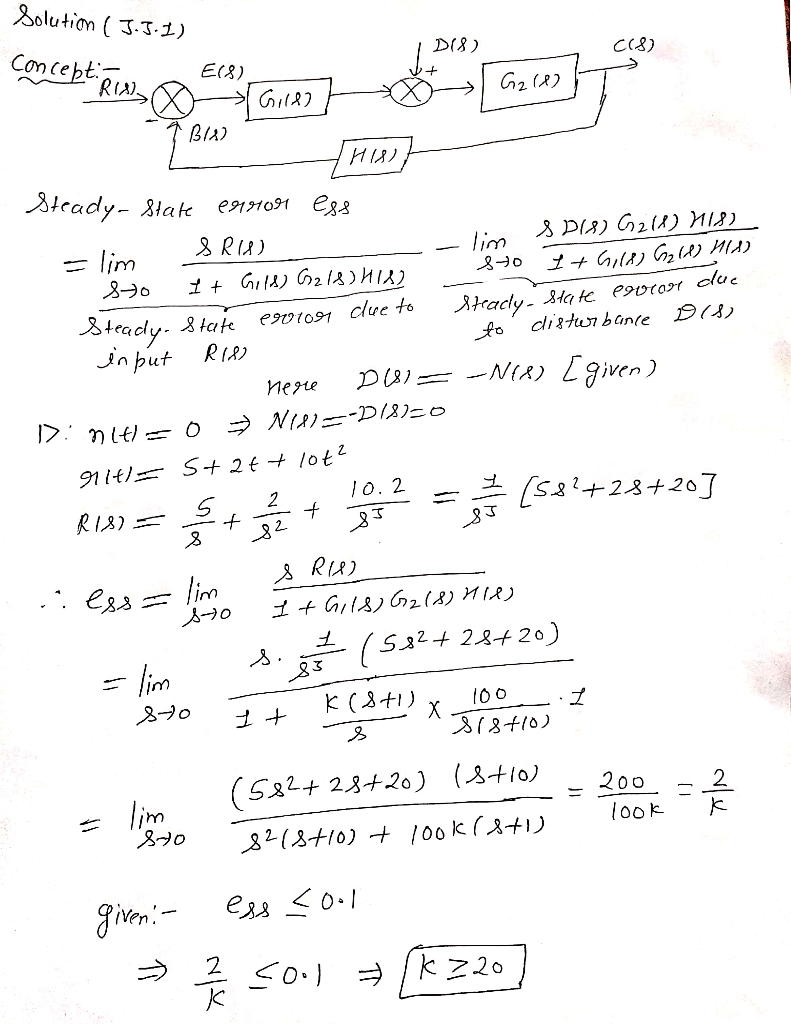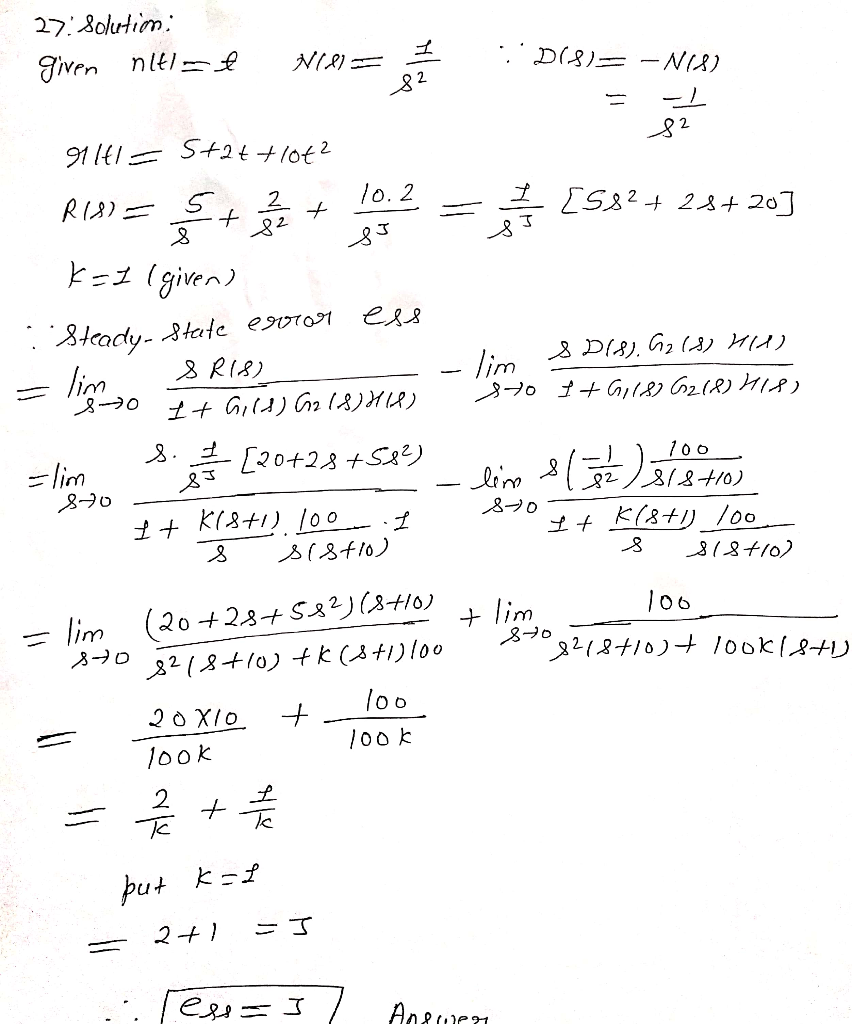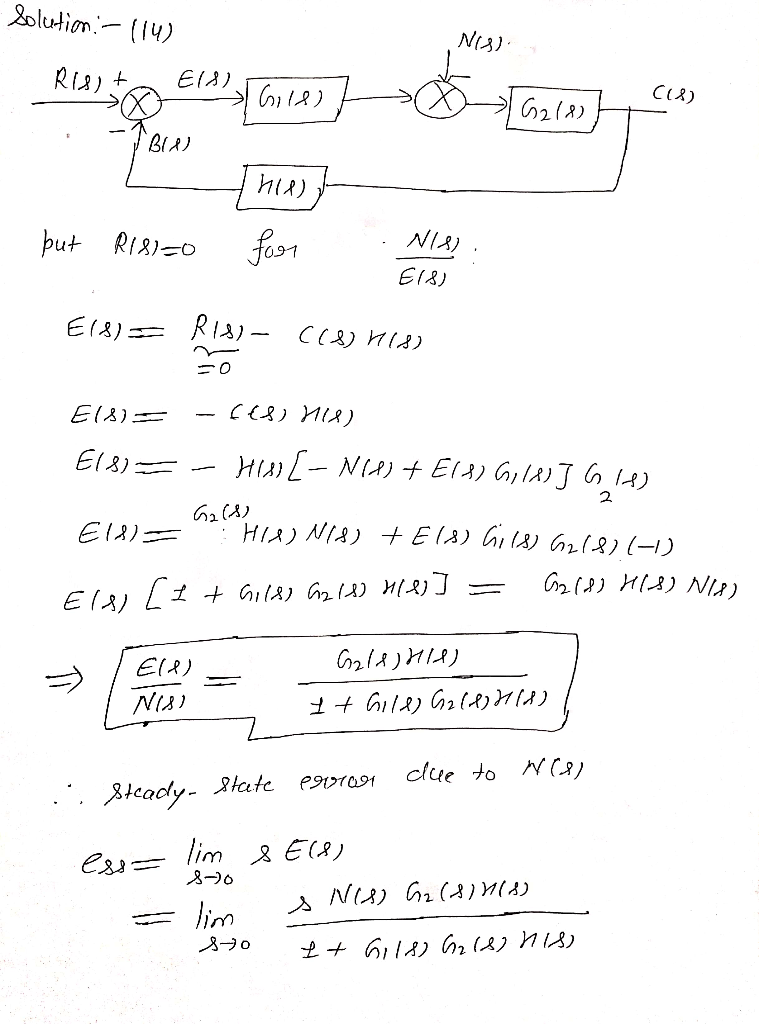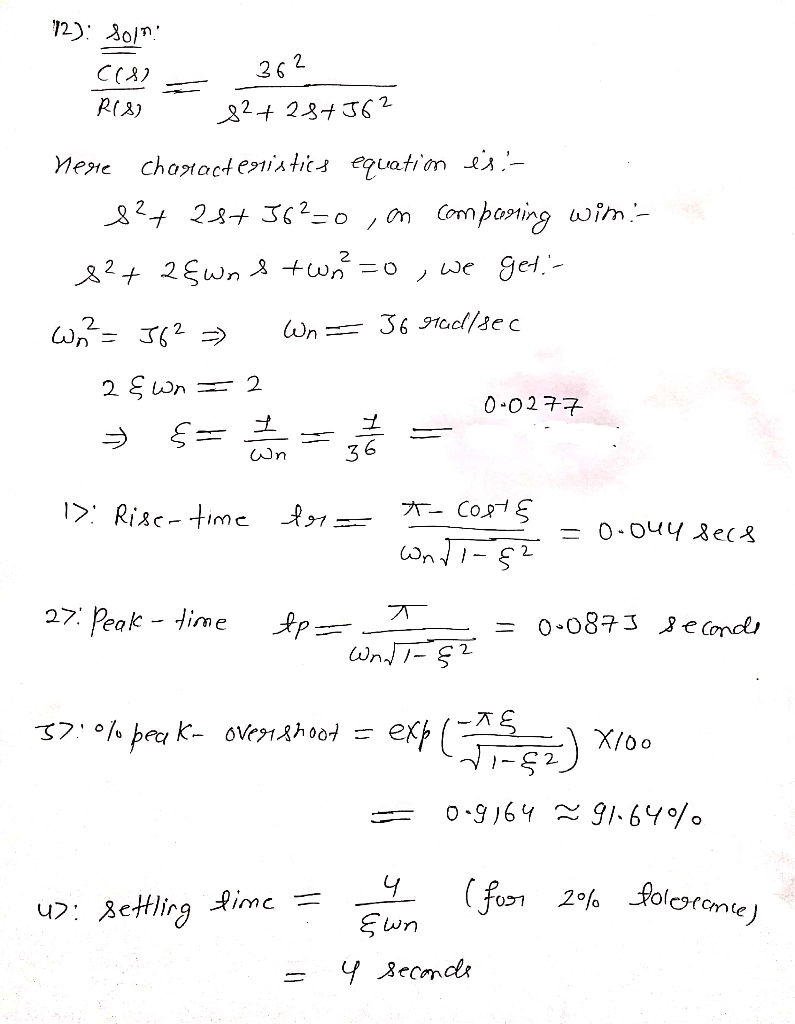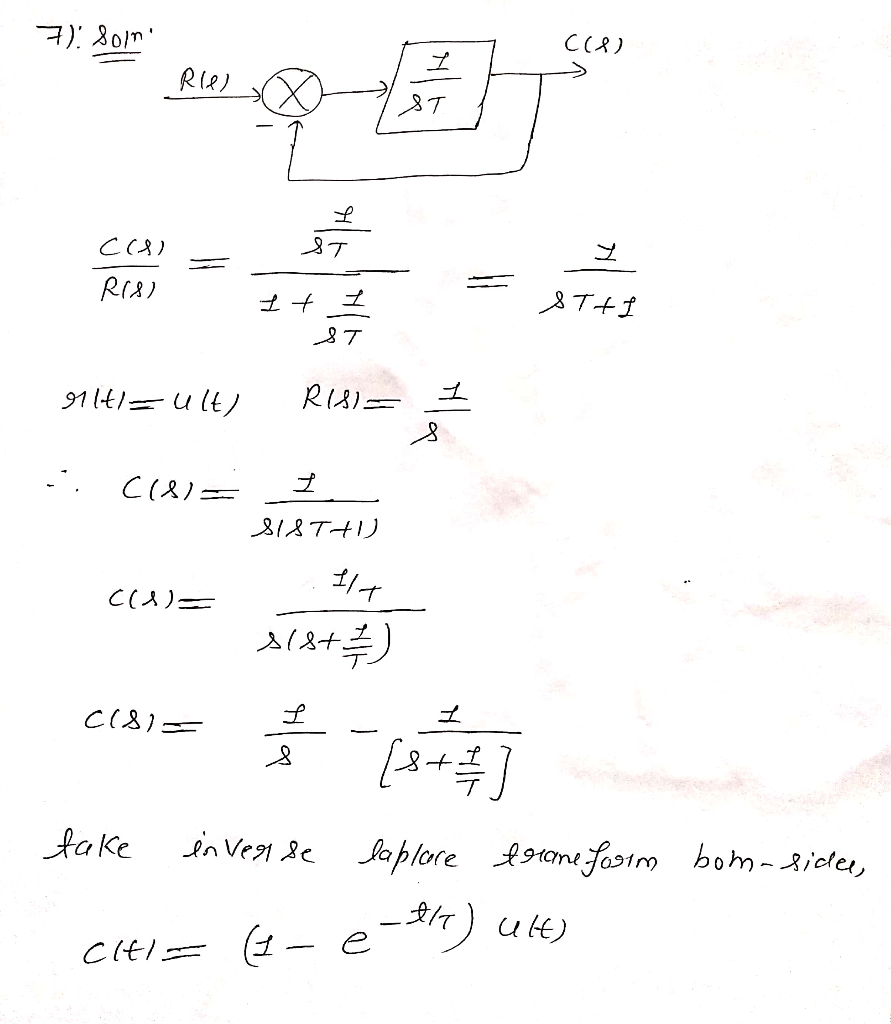#### Earn Coins

Coins can be redeemed for fabulous gifts.

Similar Homework Help Questions
• ### In the block diagram of the feedback control system shown in figure below, Gp(s) is the...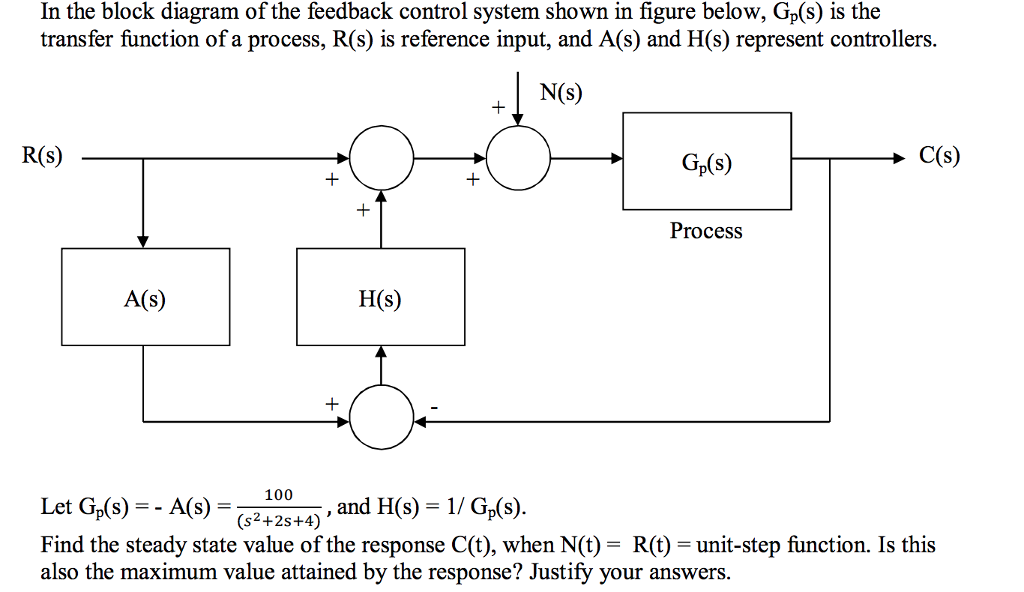In the block diagram of the feedback control system shown in figure below, Gp(s) is the transfer function of a process, R(s) is reference input, and A(s) and H(s) represent controllers. N(S) R(s) Gp(s) Process A(s) H(s) = _100_ , and H(s)-1 / GAS). Let Gs)-A(S)5.and Find the steady state value of the response C(t), when N(t) = R(t) = unit-step function. Is this also the maximum value attained by the response? Justify your answers. (s2+2s+4)

• ### 66. The system shown in Figure P6.16 has G (s) = 1/s(s+2) (s + 4). Find...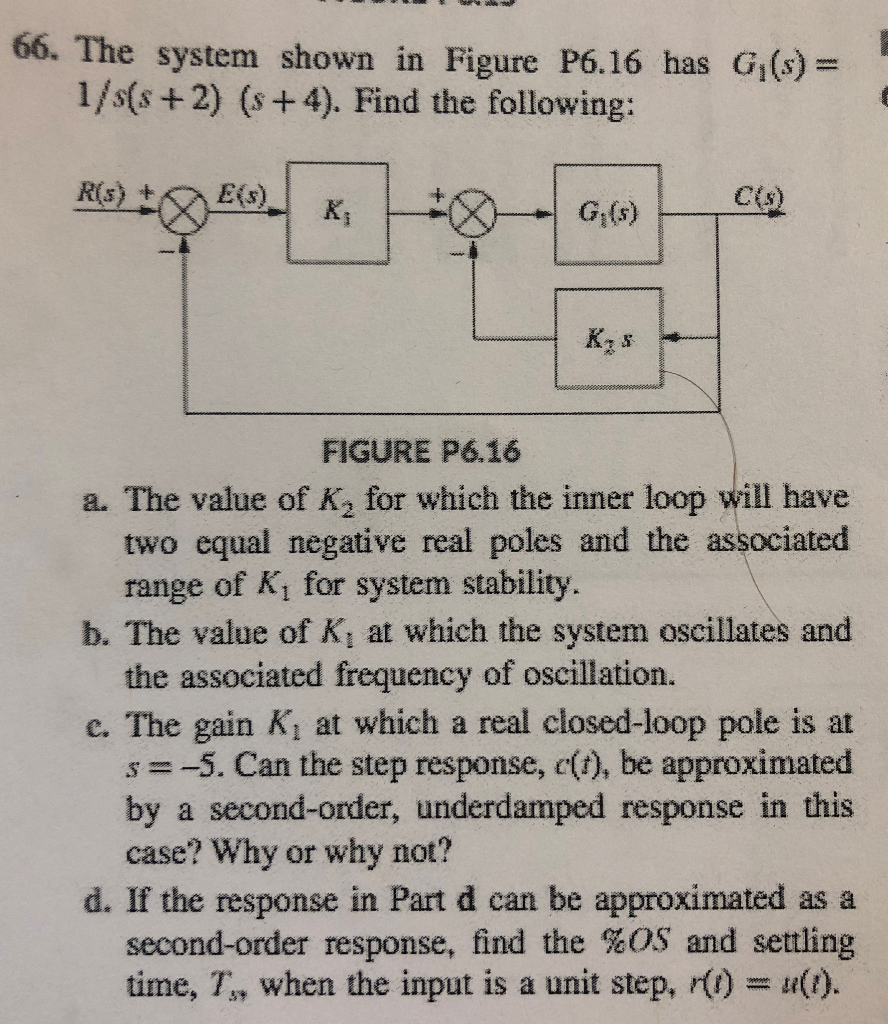66. The system shown in Figure P6.16 has G (s) = 1/s(s+2) (s + 4). Find the following: R(s) + E() K G,G) KES FIGURE P6.16 a. The value of K, for which the inner loop will have two equal negative real poles and the associated range of K, for system stability. b. The value of K, at which the system oscillates and the associated frequency of oscillation. c. The gain Ki at which a real closed-loop pole is at...

• ### C(8) for the system shown in Figure 1. R(S Find the equivalent transfer function, Geg (s)...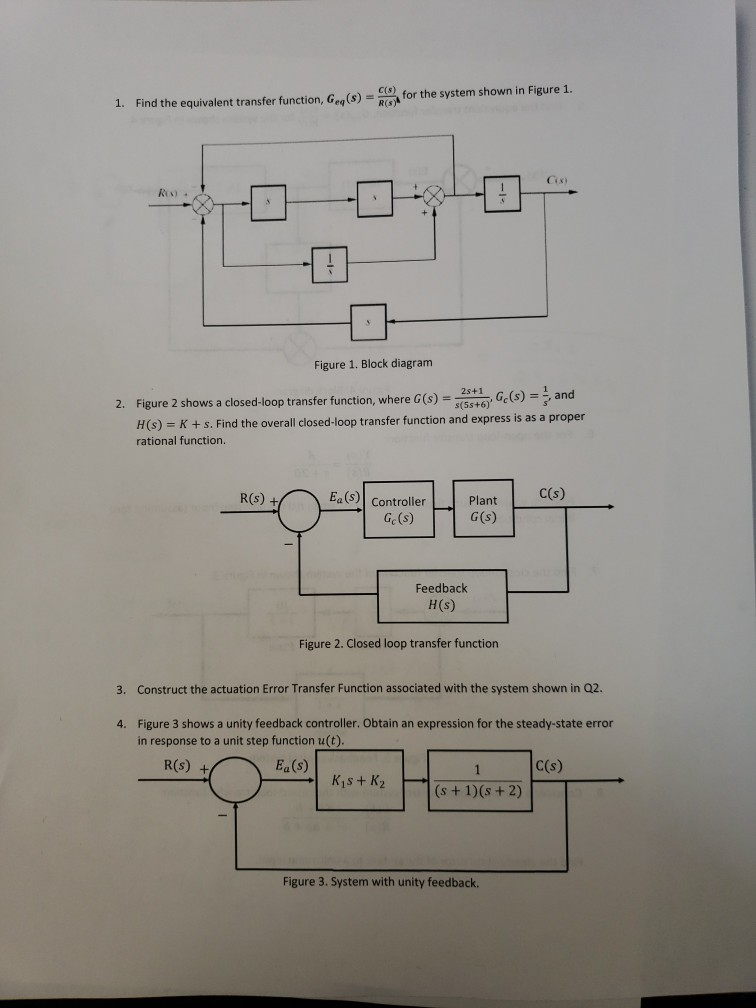C(8) for the system shown in Figure 1. R(S Find the equivalent transfer function, Geg (s) 1 Cix) Figure 1. Block diagram 2s+1 s(5s+6Ge(s) = and Figure 2 shows a closed-loop transfer function, where G(s) 2. proper H(s) K+s. Find the overall closed-loop transfer function and express is as rational function. C(s) Ea (s) Controller R(s) +/ Plant G(s) Ge (s) Feedback H(s) Figure 2. Closed loop transfer function Construct the actuation Error Transfer Function associated with the system shown...

• ### Problem 4. Consider the control system shown below with plant G(s) that has time con- stants...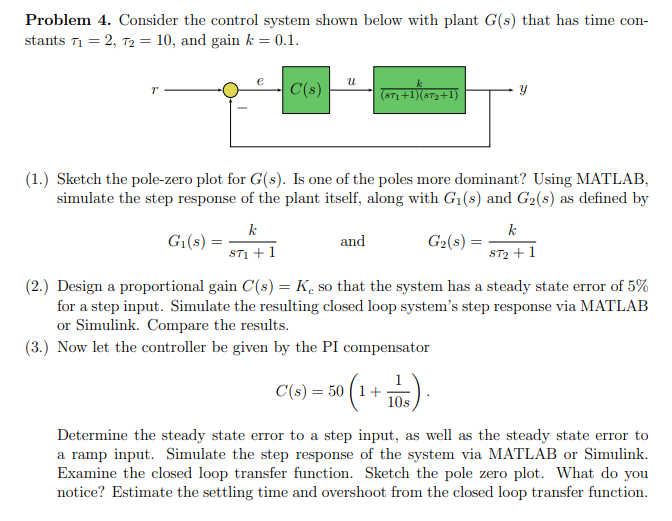Problem 4. Consider the control system shown below with plant G(s) that has time con- stants T1 = 2, T2 = 10, and gain k = 0.1. 4 673 +1679+1) (1.) Sketch the pole-zero plot for G(s). Is one of the poles more dominant? Using MATLAB, simulate the step response of the plant itself, along with G1(s) and G2(s) as defined by Gl(s) = and G2(s) = sti + 1 ST2+1 (2.) Design a proportional gain C(s) = K so...

• ### Q.4 A position control system is shown in Figure Q4. Assume that K(s) = K, the plant 50 s(0.2s +1) transfer function is given by G(s) s02s y(t) r(t) Figure Q4: Feedback control system. (a) Design a l...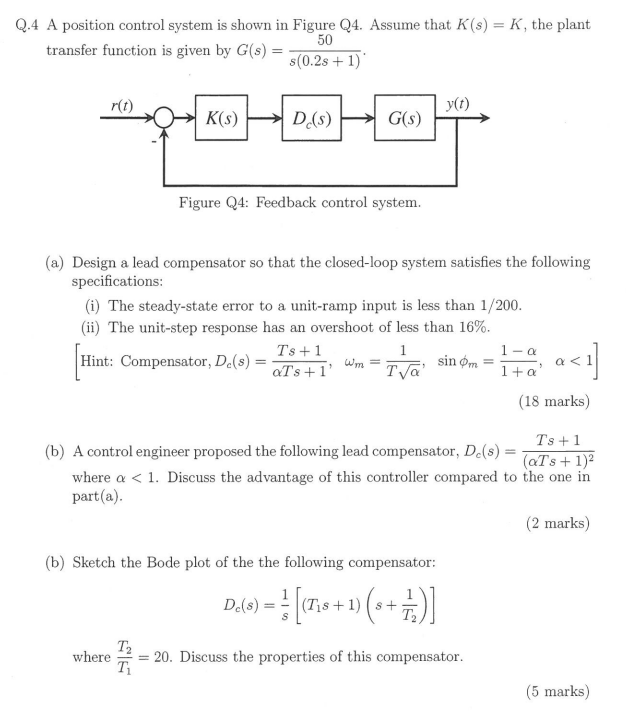Q.4 A position control system is shown in Figure Q4. Assume that K(s) = K, the plant 50 s(0.2s +1) transfer function is given by G(s) s02s y(t) r(t) Figure Q4: Feedback control system. (a) Design a lead compensator so that the closed-loop system satisfies the following specifications (i) The steady-state error to a unit-ramp input is less than 1/200 (ii) The unit-step response has an overshoot of less than 16% Ts +1 Hint: Compensator, Dc(s)=aTs+ 1, wm-T (18 marks)...

• ### 10mksl Question 1(a) C(s) G,s) H(s) Figure A3.1. System for Question 1(a) Figure A3.1 shows a...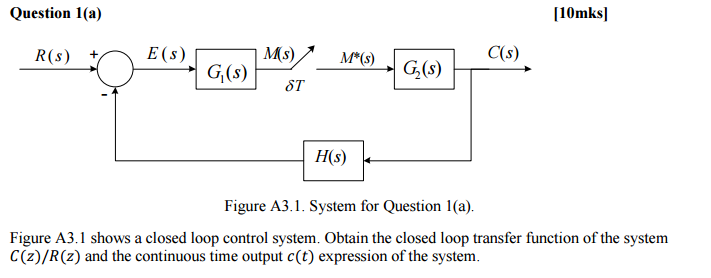10mksl Question 1(a) C(s) G,s) H(s) Figure A3.1. System for Question 1(a) Figure A3.1 shows a closed loop control system. Obtain the closed loop transfer function of the system C(z)/R(z) and the continuous time output c(t) expression of the system.

• ### Consider the following control system: R + Let G(s) s +23-3 and H(s) K where K is some positive c...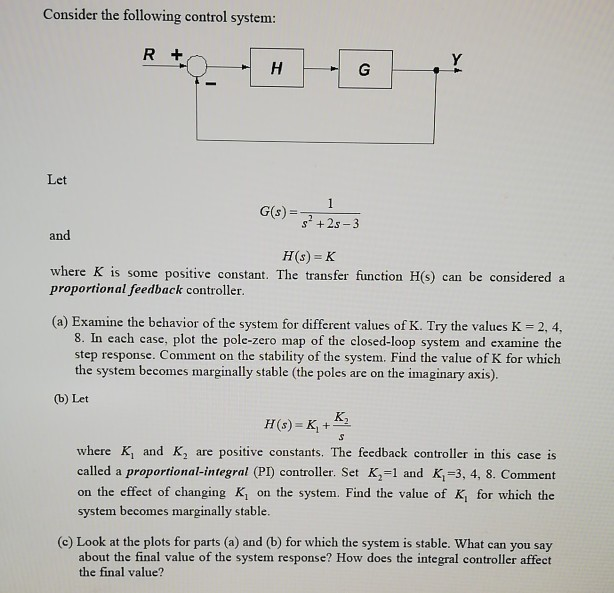Consider the following control system: R + Let G(s) s +23-3 and H(s) K where K is some positive constant. The transfer function H(s) can be considered a proportional feedback controller. (a) Examine the behavior of the system for different values of K. Try the values K 2, 4, 8. In each case, plot the pole-zero map of the closed-loop system and examine the step response. Comment on the stability of the system. Find the value of K for which...

• ### Problem B-3-19 Obtain in a closed form the response sequence c(kT) of the system shown in Figure ...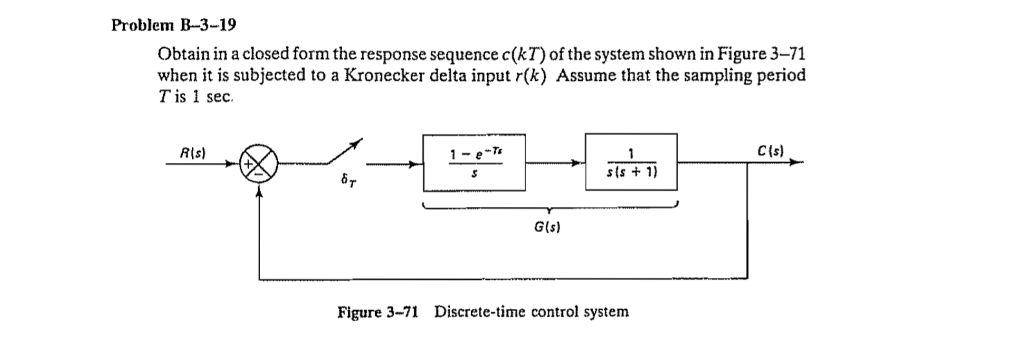Problem B-3-19 Obtain in a closed form the response sequence c(kT) of the system shown in Figure 3-71 when it is subjected to a Kronecker delta input r(k) Assume that the sampling period Tis 1 sec CIs) RIs) 1-e- 1 sis + 1) G(s) Figure 3-71 Discrete-time control system Problem B-3-19 Obtain in a closed form the response sequence c(kT) of the system shown in Figure 3-71 when it is subjected to a Kronecker delta input r(k) Assume that the...

• ### Q2 (a) Consider the control system shown in Figure Q1 (a). Obtain the closed-loop transfer function...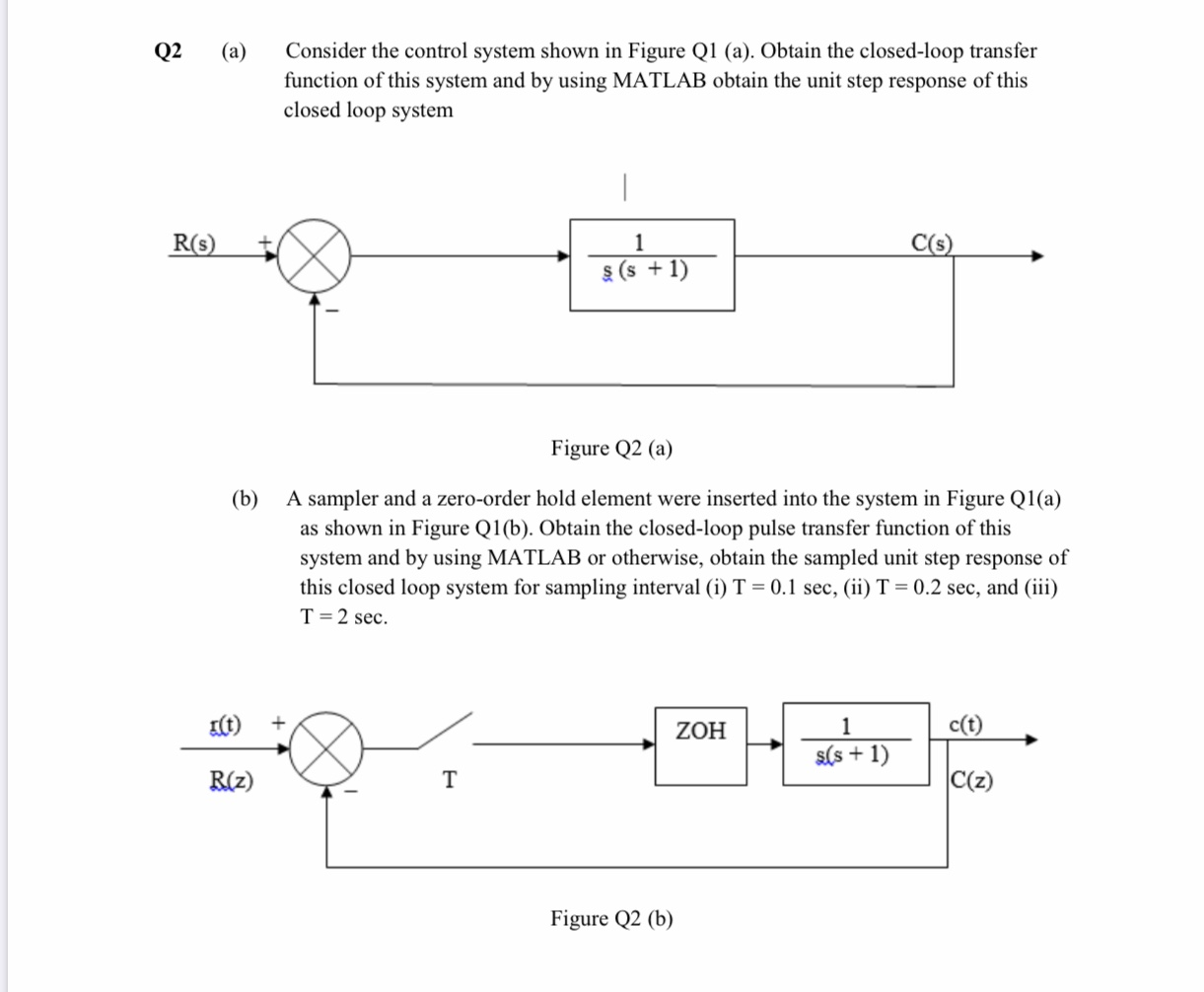Q2 (a) Consider the control system shown in Figure Q1 (a). Obtain the closed-loop transfer function of this system and by using MATLAB obtain the unit step response of this closed loop system - R(S) c(s) 36+1) (s + 1) Figure Q2 (a) (b) A sampler and a zero-order hold element were inserted into the system in Figure Q1(a) as shown in Figure Q1(b). Obtain the closed-loop pulse transfer function of this system and by using MATLAB or otherwise, obtain...

• ### Y(z) c(t), C(s) r(t), R(S) + - et), E(S) E*(s), Ez To- D(z) G (s) =...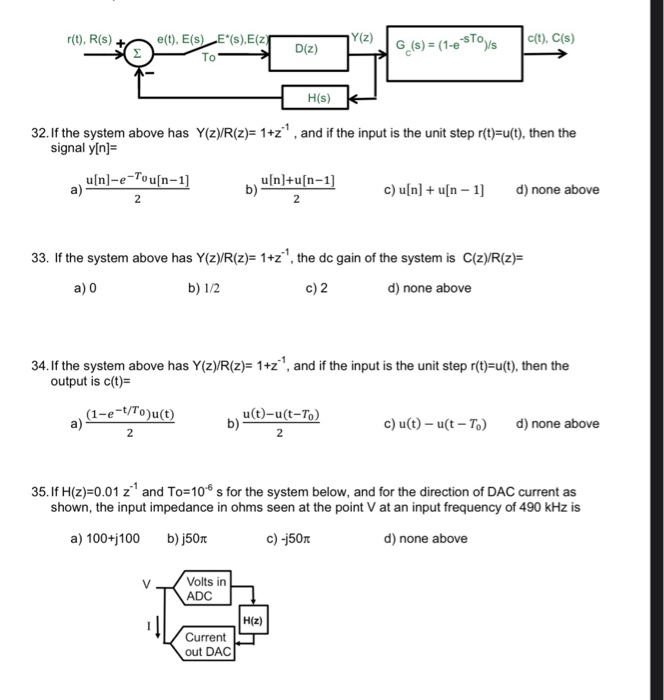Y(z) c(t), C(s) r(t), R(S) + - et), E(S) E*(s), Ez To- D(z) G (s) = (1-e-STºys H(s) 32. If the system above has Y(z)R(z)= 1+z), and if the input is the unit step r(t)=u(t), then the signal y[n]= u[n]-e-Tou[n-1) | u[n]+u[n-1] a) c) u[n] + u[n-1) d) none above 33. If the system above has Y(z)/R(z)= 1+z), the dc gain of the system is C(z)/R(z)= b) 1/2 c)2 d) none above a) o 34. If the system above has...